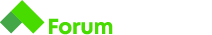# Help with Time JS Fundamentals task

Hi and good day y’all.
Can I get a little bit of help with the following task…

# Time

Write a program that reads days, hours, minutes and seconds from the standard input. Display the total amount of seconds.

this is my solution but it doesn’t get registered.

let seconds = 1;
let minute = 60 * seconds;
let hour = 60 * minute;
let day = 24 * hour;

total = (4 * day) + (3 * hour) + (11 * minute) + (40 * seconds);
print(total)

I know is not the most elegant solution but it does work.
Does anybody have a clue as to what I’m getting wrong?

Hello,

Maybe you can share the whole description of the task? I don’t really remember this particular one. The thing I see right away, the task description says you should read the time from the standard input and you are not using gets() anywhere. This way you have hardcoded the time values in your program and whatever input judge passes to you, your program returns the answer for 4 days, 3 hours, 11 minutes etc… You can use gets() to take the input out for each individual test. For example, if your input is one string with all the data you can do it like this - let time = gets(). If it’s a bunch of rows with the different hours, minutes, second you can do it like:
let hours = gets()
let minutes = gets()
and etc.
This will take out the values for each test judge passes you, save them in your declared variables and pass them on to your logic.

P.S. You can use a plus in front of gets - +(gets()) to parse it immediately to a number if you are working with numbers.

Sorry, I didn’t pasted the whole description…

# Time

Write a program that reads days, hours, minutes and seconds from the standard input. Display the total amount of seconds.

## Input

• First line - d - days
• Second line - h - hours
• Third line - m - minutes
• Fourth line - s - seconds

## Output

• On the only line of output, print the total amount of seconds

## Constraints

• 0 <= d, h, m, s <= 1000

## Input

``````4
3
11
40
``````

## Output

``````357100
``````

## Input

``````0
2
6
0
``````

## Output

``````7560
``````

I tried with the gets but i got no luck.
I’ll leave it for another day I guess.

But thanks for the response

Hello,

As you can see from the input, there are 4 lines which means you need to call gets() 4 times in order to get all the data. Here is what you can do:

let days = gets();
let hours = gets();
let minutes = gets();
let seconds = gets();

In that way, you will have all 4 inputs and you can start implementing your logic. Do not forget to use print() instead of console.log() at the end. Good luck!
Note: there are constraints so think of a way to implement that as well1 Like